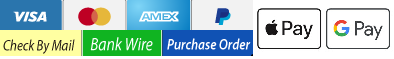### How Many Tables Fit in a Room? Calculate by Square Footage

How many square feet do you have?(Do not use commas, input numbers only)
﻿

#### Based on 12 Square Feet Per Person

Table Type# of tables# of people
72 Round--
60 Round--
48 Round--
42 Round--
36 Round--
8 Foot Rectangle--
6 Foot Rectangle--
4 Foot Rectangle--

#### Based on 10 Square Feet Per Person

Table Type# of tables# of people
72 Round--
60 Round--
48 Round--
42 Round--
36 Round--
8 Foot Rectangle--
6 Foot Rectangle--
4 Foot Rectangle--

# How Many Tables Fit in a Room? Calculate by Square Footage

Instructions

Just input your square footage, and the result will be 2 scenarios. The first is based on 12 square foot per person, and the 2nd is based on 10.

Input "numerals" only, no commas etc.

Common Questions This Calculator will Answer

How many 72, 60, 48, and 36 inch round tables will fit in a room?
How many 8 foot, 6 foot, and 4 foot rectangle tables will fit in a room?

All you need to know is the square foot of the room. You can measure square footage of a room by multiplying the width x length of the room. Remember to subtract any square footage that tables cannot occupy, like a dance floor, bar, server station, etc.

This calculator uses the following table dimensions.

30 x 48

30 x 72

30 x 96

4 Foot Round

5 Foot Round

6 Foot Round

The calculator rounds down when the result has a fraction of a table.

Other resources866-677-4227

Mon-Fri 8am-5pm EST